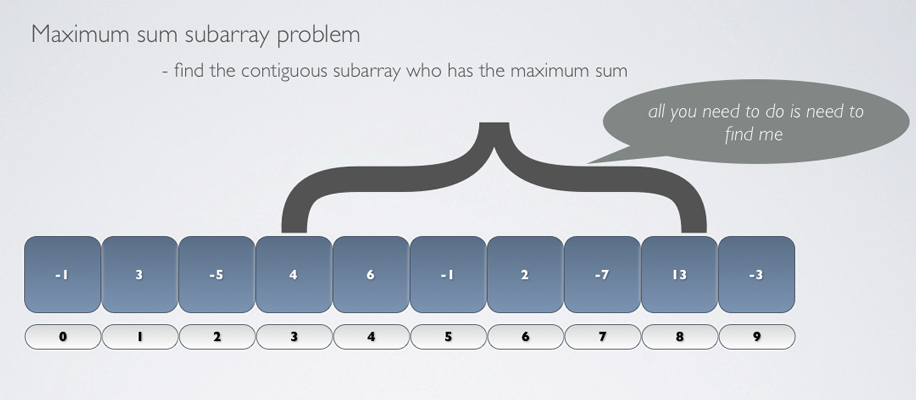Update : Previous version of the code failed for some inputs, as pointed out by @Thinker in the first comment. Changes to the program and the presentation were made. Tested to satisfy most conditions that I could google for.

In a desperate attempt to increase my sad looking stackoverflow reputation, I replied to an old but interesting problem.

The problem goes like this :

Given `Random integers in array. Find the greatest sum of a continuous subset`

The problem is otherwise known as the Maximum subarray problem and is beaten to death numerous times.So, instead of just translating a Python program into Java, I decided to pull together some slides which explains how this algorithm works.

And to bore you to death, here is the Java version of the modified algorithm :

``````package me.rerun;

public static void main(String[] args) {
int[] intArr={3, -1, -1, -1, -1, -1, 2, 0, 0, 0 };
//int[] intArr = {-1, 3, -5, 4, 6, -1, 2, -7, 13, -3};
//int[] intArr={-6,-2,-3,-4,-1,-5,-5};
findMaxSubArray(intArr);
}

public static void findMaxSubArray(int[] inputArray){

int maxStartIndex=0;
int maxEndIndex=0;
int maxSum = Integer.MIN_VALUE;

int cumulativeSum= 0;
int maxStartIndexUntilNow=0;

for (int currentIndex = 0; currentIndex < inputArray.length; currentIndex++) {

int eachArrayItem = inputArray[currentIndex];

cumulativeSum+=eachArrayItem;

if(cumulativeSum>maxSum){
maxSum = cumulativeSum;
maxStartIndex=maxStartIndexUntilNow;
maxEndIndex = currentIndex;
}
else if (cumulativeSum<0){
maxStartIndexUntilNow=currentIndex+1;
cumulativeSum=0;
}
}

System.out.println("Max sum         : "+maxSum);
System.out.println("Max start index : "+maxStartIndex);
System.out.println("Max end index   : "+maxEndIndex);
}

}
``````

Rajesh was kind enough to write the implementation in Scala. Sweet huh?